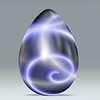Regular slog for base sqrt(2) - Using z=2IvarsLong Time FellowPosts: 366 Threads: 26 Joined: Oct 2007 11/25/2007, 09:05 AM (This post was last modified: 11/25/2007, 09:07 AM by Ivars.) Amazing fractals. So is there now final formula for all branches of h(z) = e^-Wk(-ln(z))? So easy so I can use Wolfram function evaluation web page? Would it work also for analytic continuation of h(z) in the range z>e^1/e and or z<0? (by using ln(-x)= ln(x) +- pi*I*(2k+1), k>=0)? IvarsjaydfoxLong Time FellowPosts: 440 Threads: 31 Joined: Aug 2007 11/26/2007, 07:09 PM (This post was last modified: 11/26/2007, 07:53 PM by jaydfox.) bo198214 Wrote:How can one find the singularities of $f^{\circ t}(x)$ and the complex fixed points of $f(x)=\sqrt{2}^x$ in your drawing? Actually, the easiest way is to look at the definition of the rslog itself. We know the two primary singularities are at 2 and 4. Therefore, we can find one tier of "images" of these two singularities with: $\log_{\sqrt{2}}(p)+\frac{2\pi k i}{\ln(\sqrt{2})},\ p \in \{2, 4\},\ \ k \in \mathbb{Z}$ We can find the next tier of images by simply repeating this process: every branch of two iterated logarithms: $\log_{\sqrt{2}}\left(\log_{\sqrt{2}}(p)+\frac{2\pi j i}{\ln(\sqrt{2})}\left)+\frac{2\pi k i}{\ln(\sqrt{2})},\ p \in \{2, 4\},\ \ j, k \in \mathbb{Z}$ This can be repeated indefinitely. Indeed, going back to the definition of the rslog, if we look at a solution for a finite n, then we can only repeat the process n times, and only for the images of the fixed point at 2. The fixed point at 4 is only a singularity when n goes to infinity. And looking at j, k, etc., we can see that setting the innermost branches to 0 allows us to generalize a shorter sequence with the infinite sequence. We can then specify a singularity by indicating if its an image of the upper or lower fixed point, and then specify its infinite sequence of branch choices, starting from the outermost, with the sequence truncated if the branch choices are all 0 beyond a certain iterated logarithm. ~ Jay Daniel FoxandydudeLong Time FellowPosts: 510 Threads: 44 Joined: Aug 2007 11/27/2007, 07:10 PM jaydfox Wrote:It's not 18*I, it's $\frac{2\pi i}{\ln\left(\sqrt{2}\right)}$ Right, sorry, thanks.GottfriedUltimate FellowPosts: 900 Threads: 130 Joined: Aug 2007 03/10/2010, 12:47 PM (This post was last modified: 03/10/2010, 12:48 PM by Gottfried.) (11/27/2007, 07:10 PM)andydude Wrote: jaydfox Wrote:It's not 18*I, it's $\frac{2\pi i}{\ln\left(\sqrt{2}\right)}$ Right, sorry, thanks. If one uses other bases in the interval 1

 Possibly Related Threads… Thread Author Replies Views Last Post [2sinh] exp(x) - exp( - (e-1) x), Low Base Constant (LBC) 1.5056377.. tommy1729 3 147 04/30/2023, 01:22 AM Last Post: tommy1729 Computing sqrt 2 with rational functions. tommy1729 0 126 03/31/2023, 11:49 AM Last Post: tommy1729 sqrt thingy at MSE tommy1729 3 707 08/14/2022, 05:44 AM Last Post: JmsNxnE^^.5 and Slog(e,.5) Catullus 7 1,269 07/22/2022, 02:20 AM Last Post: MphLeeSlog(Exponential Factorial(x)) Catullus 19 3,415 07/13/2022, 02:38 AM Last Post: CatullusSlog(x^^^2) Catullus 1 545 07/10/2022, 04:40 AM Last Post: JmsNxn Base -1 marraco 15 20,458 07/06/2022, 09:37 AM Last Post: Catullus I thought I'd take a crack at base = 1/2 JmsNxn 9 3,147 06/20/2022, 08:28 AM Last Post: CatullusSlog(e4) Catullus 0 511 06/16/2022, 03:27 AM Last Post: Catullus sqrt(!) and sqrt(exp) Kouznetsov 4 9,734 06/08/2022, 05:32 AM Last Post: Catullus

Users browsing this thread: 1 Guest(s)